Resonant behavior of stochastic oscillations of general relativistic disks driven by a memory-damped friction*
School of Physics and Electronic Engineering, Hubei University of Arts and Science, Xiangyang 441053, China

Corresponding author. E-mail: wzy425@126.com

*Project supported by the National Natural Science Foundation of China (Grant No. 11045004) and the Key Program of the Scientific Research Foundation of the Education Bureau of Hubei Province, China (Grant No. D20132603).

Abstract

By using a generalized Langevin equation to describe the vertical oscillations of a general relativistic disk subjected to a memory-damped friction and a stochastic force, we derive the power spectrum density (PSD) of accretion disk oscillating luminosity by the method of Laplace transform, and discuss the influence of the system parameters on the resonant behavior in PSD curves. The results show that as the damping strength α and memory time τ of the friction increase, the variation of PSD with spectrum frequency f from monotonous decreasing to occurring maximums, and the phenomenon of a general stochastic resonance (SR) with a single peak and multi-peaks can be found in PSD curves. The radial distance parameter n, the mass M, and spin parameter a* of the black hole determine the inherent frequency of vertical oscillations in the disk, and they have significant influences on the SR phenomena in a system of black hole binaries.

Keyword: 98.62.Mw; 97.10.Sj; 97.60.Lf; accretion disk; power spectrum density; memory-damped friction; stochastic resonance
1. Introduction

It is well known that the accretion disk has been an important ingredient in our current understanding of many astrophysical systems on all scales, and the accretion disks around compact objects have become standard models for studying many astrophysical phenomena. Because the accretion of matters on to compact objects can produce high-energy radiation, accretion disks, as a powerful and interesting theoretical model, is widely believed to be the source of energy in various astronomical objects such as quasars, black hole x-ray binaries, and active galactic nuclei (AGNs).[1, 2] The physics of accretion disks has been used to explain a number of observations of them. However, one of the most prominent observational features of the black hole binaries and AGNs is the strong and chaotic time variations in x-rays. For example, quasi-periodic oscillations (QPOs) are occasionally observed. A lot of disk oscillation models have been proposed to explain the reason of such variability. For example, the normal-mode oscillations in geometrically thin disks have been studied extensively both within Newtonian gravity and the relativistic framework. However, an accretion disk may be subjected to stochastic force of large scales, which represents either the internal force of the disk by the nonlinear terms or the interaction with an external medium, such as tidal force, shock wave, outbursts, friction force, and so on.[12, 13] By taking into account the presence of a viscous dissipation and a stochastic force, the vertical oscillations of accretion disks were described by a Langevin equation.[14, 15] Then, the vertical displacements, velocities and the luminosities of stochastically perturbed disks in both the static Schwarzschild and rotating Kerr geometries were obtained and were used to explain the intra-day variability of AGNs.[16, 17] Using a Langevin equation to describe the vertical oscillations of a geometrically thin disk around a black hole, we investigated the luminosity and power spectrum density (PSD) both in the non-relativistic and relativistic cases, and discussed the stochastic resonance (SR) phenomenon in PSD curves and found that the simulated PSD curves of luminosity for disk oscillations have the same profile as the observed PSD of black hole x-ray binaries, and the SR may be an alternative interpretation of the low-frequency QPOs (LFQPOs). However, those models could not explain the high-frequency QPOs (HFQPOs) because the resonance frequency is generally less than 1, and the Langevin equation in those modes provides a statistical description of the Brownian motion only in the long time limit, for long times as compared with the characteristic relaxation time of the velocity autocorrelation function.[15, 21, 22] It could also not describe the dynamics of a homogeneous system without restriction on a time scale. By considering a general memory and the retarded effects of frictional force, Harko et al. used a generalized Langevin equation with colored noise and fluctuation-dissipation theorems to describe the stochastic oscillation of the general relativistic accretion disks[15, 16] and found that the memory effects modify the dynamical behavior of the disk, as well as the energy dissipation processes.

In this paper, we extend the analysis of our research in Ref.  based on the model given by Harko in Ref. . By introducing a systematic force with an integral kernel instead of the simple friction term, we use a generalized Langevin equation to describe the stochastic oscillations of the particles in accretion disks around the black hole in a relativistic framework. Then, we investigate the PSD of oscillating luminosity, and discuss the resonant behaviors in PSD curves.

2. Generalized Langevin equation and the PSD for stochastic oscillations of accretion disks

We consider a thin accretion disk around a black hole in contact with an isotropic and homogeneous external heat bath. Taking into account the retarded effects of the frictional force, the vertical oscillation of the particles in this stochastically perturbed disk can be described by a generalized relativistic Langevin equation, given by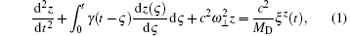where z and ω are the displacement and frequency of particles’ vertical oscillation; MD and c express the mass of the accretion disk and the speed of light in a vacuum, respectively. The damping kernel function is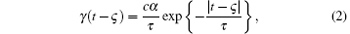and the autocorrelation function of the stochastic force satisfies the condition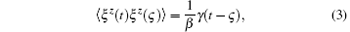where β is a constant, τ represents a characteristic disk memory time, and α is interpreted as the disk damping strength, which describes the strength of energy dissipation in the disk. By introducing a set of dimensionless parameters (θ , σ , Z) defined as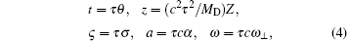equation (1) can be changed to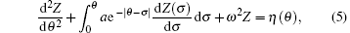where η (θ ) satisfies the following statistical properties: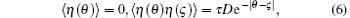where D = /β .

For a Kerr accretion disk, by denoting the radial distance ρ in terms of the gravitation radius and the mass M of the black hole, that is ρ = nGM/c2, n = constant (n ≥ 6). By setting a* = J/M2 = a/M (a* ∈ [0, 1]) representing the black hole spin and denote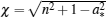, the expression of ω can be represented as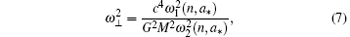where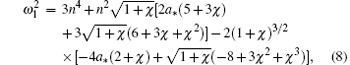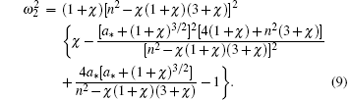We assume that the dimensionless initial displacement and velocity of disk oscillations are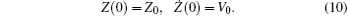Using the method of Laplace transform, we can obtain the expressions of Z(θ ), Ż (θ ), and (θ ) by solving Eq. (5) as follows: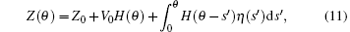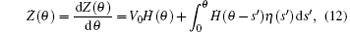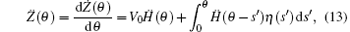where (θ ) = d(θ )/dθ = d2H(θ )/dθ 2. Here, H(θ ) is the response function, and its Laplace transform is given by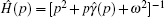,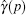is the Laplace transform of the memory damping kernel γ (θ ) and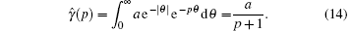When a and ω satisfy the following condition: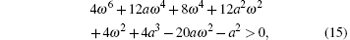the solution of H can be represented in the form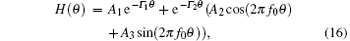where the coefficients A1, A2, and A3 are constants of integration determined by initial conditions and system parameters; Γ 1, Γ 2, and f0 are the set of roots of the algebraic equation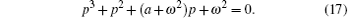The total energy of the oscillating disk is the sum of kinetic plus potential energy, which can be written as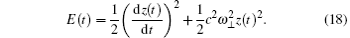The time variation of the energy express output luminosity L, which represents the energy loss of the disk due to viscous dissipation and the presence of stochastic force, can be written in dimensionless form as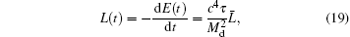where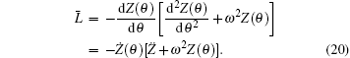The steady-state mean autocorrelation function of dimensionless luminosity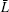can be described as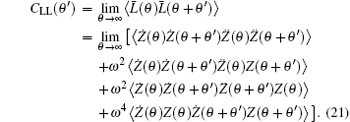The autocorrelation function CLL(θ ′ ) is a measure of correlation between luminosities at time θ and θ + θ ′ , and it is an important physical quantity to characterize the dynamic behavior of stochastic systems in a nonequilibrium steady state. The output power spectra and normalized mean luminosity fluctuation can be derived from it. According to Eq. (19), we can see that the correlation of the memory friction and stochastic force is an important factor of dynamical behavior for the vertical oscillations of the disk described by Eq. (1).

By Fourier transform, equation (21) may be translated into the PSD (P(f)) of oscillating luminosity of the accretion disk as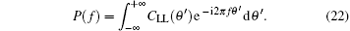3. Resonant behavior in the PSD of oscillating luminosity of accretion disk

According to the above discussion, we find that, when the physical parameters take certain values, the displacement and velocity of vertical oscillations of an accretion disk can be solved by the method of Laplace transform. Then we may obtain the PSD of the disk oscillating luminosity in both static Schwarzschild geometry and rotating Kerr geometry, respectively. It is shown that different forms of general SR exist in curves of the PSD versus spectrum frequency, described as follows.

3.1. Variation of the PSD with memory time τ

Figure 1 depicts the PSD curves with taking the memory time τ of damping kernel function as a parameter. It can be found that the continuum of PSD is approximated as a broken power law, and the power-law index keeps constant in two different ranges of spectrum frequency f, and different SR phenomena occur when τ takes different values as follows. (i) In the case of τ = 0.0001, the PSD is monotonically descended with the increase of f. (ii) For τ = 0.001, a maximum presents in the PSD curve, which is not monotonic. This is a general SR phenomenon. (iii) When τ = 0.01, the PSD curve shows three-peak resonance, with nonmonotonic behavior for the frequencies f close to three resonance frequencies, which is a typical multi-peaks SR. The resonance peak corresponding to the highest frequency is more sharp and higher than the other two peaks, so its resonance is more pronounced. Two peaks corresponding to the lower f are very close together, and their heights are nearly equal. (iv) When τ increases to 0.1, however, the number of resonance peaks in the PSD curves decreases to two, it is a double-peak SR. We can also find that as the memory time τ increases, the SR phenomenon is more obvious, and the position of peaks turns toward the increasing f.

 Figure Option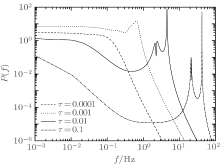Fig. 1. The PSD of the luminosity for a stochastically oscillating disk around a Kerr black hole with the mass M = 10Msun and a* = 0.9 and for n = 7, α = 10− 7.

Above results illustrate that the form of SR in PSD curves of the accretion disk oscillating is significantly induced by the memory time τ of friction. Therefore, in contrast to a general relativistic disk with fixed damping, the vertical oscillation of an accretion disk driven by a memory-damped friction has more rich resonant behavior, namely, there is a multi-peak resonance. We consider that the memory time has an essential influence on the SR phenomenon in PSD curves, and the reason for this may be that the prolonged memory makes more energy produced by stochastic force transforming into luminosity by viscous dissipation, and induces resonance, which appears easier.

3.2. Variation of the PSD with disk damping strength α

The PSD as a function of spectrum frequency f for different values of the disk damping strength α and memory time τ are plotted in Fig. 2. From Fig. 2(a), we can clearly see that, when τ takes the value of 0.0001, the PSD decreases monotonically with f in the case of α = 10− 6, whereas, in the case of α > 10− 5, the PSD curves exhibit double-peak resonance, of which two peaks have the same height, and the peak corresponding to the higher frequency is sharper. As α increases, the value of the PSD is decreased, but the resonance peaks are more sharp, and their positions move towards the increased f, the resonance is more pronounced too.

 Figure Option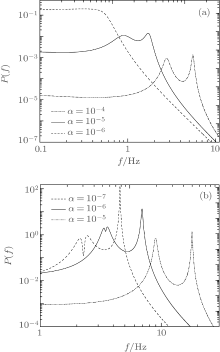Fig. 2. The PSD of the luminosity for a stochastically oscillating disk around a Kerr black hole with the mass M = 10Msun, a* = 0.9, and n = 7. (a) τ = 0.0001, (b) τ = 0.01.

The influence of damping strength α on the SR in PSD curves in the case of τ = 0.01 is shown in Fig. 2(b). When α takes a small value, there are three resonance peaks, which show the same tendency as in the corresponding part of Fig. 1. With the increase in α , two peaks corresponding to the lower f are close to each other until they merge into one resonance peak, at the same time, the well between them continues to rise until it disappears, finally presenting a double- peak SR as shown in the case of α = 10− 5.

The results mentioned above show that both the damping strength α and memory time τ of friction have the same influence on the SR in the PSD curves of accretion disk oscillating luminosity. According to Eqs. (3) and (4), we can find that the strength of friction and stochastic force are increased as α increases, they can induce the resonant behavior in PSD curves to be more pronounced. In addition, the influences of α on the position and intensity of the resonance peak are greatly different from the case of fixed damping friction shown in Ref. , of which damping strength only influences the height rather than the position of the resonance peak.

3.3. Variation of the PSD with the radial distance parameter n, mass M and spin parameter a* of the black hole

In order to discuss the influences of the system parameters for black hole binaries on PSD, we plot the curves of the output luminosity PSD for different values of the radial distance parameter n, the mass M, and spin parameter a* of the black hole shown in Fig. 3. These graphs show the same double-peak or three-peak resonances as shown in Fig. 2. The following two points are worth pointing out. (i) Both M and n have the same influence on the PSD. On the one hand, two peaks corresponding to the lower f are close to each other as n or M decrease, but the well between them continues to deepen. On the other hand, with the decrease in n and M, the resonance peaks become higher and sharper, and their positions turn towards the increasing f. (ii) The spin parameter a* has little influence on the fully PSD curve, for the SR phenomenon, it only makes the positions of peaks change.

 Figure Option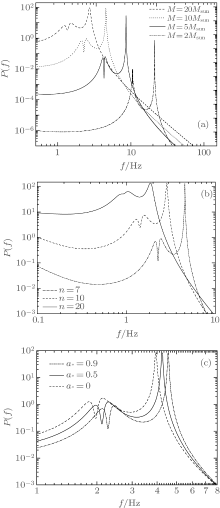Fig. 3. The PSD of the luminosity for a stochastically oscillating disk around a black hole for τ = 0.01, α = 10− 7, and (a) a* = 0.9, n = 7, (b) a* = 0.9, M = 10Msun, (c) n = 7, M = 10Msun.

According to Eqs. (7)– (9), the inherent frequency of vertical oscillations of an accretion disk ω is determined with the three parameters mentioned above. So we can see that as ω is increased, the resonance frequency is higher, and the resonant behavior in the PSD curve of disk oscillating luminosity is more pronounced.

4. Conclusion

In this work, considering a generalized Langevin equation with a damping kernel function and a stochastic term to describe the vertical oscillations of a general relativistic disk around a black hole, we derived the PSD of disk oscillating luminosity, and investigated the resonant behavior in PSD curves. The main results show that there are two different forms of SR observed in PSD curves for different parameter regimes. When the parameters of α and τ are small, the PSD is monotonically decreased with spectrum frequency f. However, as α and τ increase, the maximums present in PSD curves, and the number changes from one to three and then decreases to two, that is, there exist single-peak and multi-peak SR phenomena, and the resonance frequency is increased with α and τ increasing. The mass M, radial distance parameter n, and spin parameter a* of the black hole have different influences on the SR phenomena because they determine the inherent frequency of vertical oscillations of the accretion disk.

Finally, it is remarkable that, in contrast to the results of a general relativistic disk with fixed damping discussed in Ref. , the PSD curves of disk oscillating luminosity not only show multi-peak SR, but also the resonance frequency can be improved to dozens of Hz. These characteristics are consistent with HFQPOs observed in black hole x-ray binaries. This result motivates us to fit the observed HFQPOs by the SR of general relativistic disks driven by memory-damped friction in our future work.

Reference
 1 Shakura N I and Sunyaev R A 1973 Astron. Astrophys. 24 337 [Cited within:1] [JCR: 5.084] 2 Lynden-Bell D and Pringle J E 1974 Mon. Not. R. Astron. Soc. 168 603 DOI:10.1093/mnras/168.3.603 [Cited within:1] [JCR: 5.521] 3 Remillard R A and Mcclintoc J E 2006 Astron. Astrophys. 44 49 DOI:10.1146/annurev.astro.44.051905.092532 [Cited within:1] [JCR: 5.084] 4 Mcclintoc J E and Remillard R A 2006 Black Hole Binaries Cambridge Cambridge Univ. Press 1 46 [Cited within:1] 5 Belloni T M, Sanna A and Méndez M 2012 Mon. Not. R. Astron. Soc. 426 1701 DOI:10.1111/j.1365-2966.2012.21634.x [Cited within:1] [JCR: 5.521] 6 Kato S 2001 Publ. Astron. Soc. Jpn. 53 1 DOI:10.1093/pasj/53.1.1 [Cited within:1] [JCR: 2.439] 7 Abramowicz M A and Fragile P C 2013 Living Rev. Relativity 16 1 DOI:10.12942/lrr-2013-1 [Cited within:1] [JCR: 22.333] 8 Titarchuk L and Osherorich V 2000 Ap. J. 542 L111 DOI:10.1086/312935 [Cited within:1] [JCR: 0.274] 9 Blaes O M, Arras P and Fragile P C 2006 Mon. Not. R. Astron. Soc. 369 1235 DOI:10.1111/j.1365-2966.2006.10370.x [Cited within:1] [JCR: 5.521] 10 Kato S 1990 Publ. Astron. Soc. Jpn. 42 99 [Cited within:1] [JCR: 2.439] 11 Kato S 2008 Publ. Astron. Soc. Jpn. 60 111 DOI:10.1093/pasj/60.1.111 [Cited within:1] [JCR: 2.439] 12 Ioannou P J and Kakouris A 2001 Ap. J. 550 931 DOI:10.1086/apj.2001.550.issue-2 [Cited within:1] [JCR: 0.274] 13 Das M K, Chaudhary A K and Tavakol R K 1996 Ap. J. 463 694 DOI:10.1086/177283 [Cited within:1] [JCR: 0.274] 14 Harko T and Mocanu G 2012 Mon. Not. R. Astron. Soc. 421 3102 DOI:10.1111/mnr.2012.421.issue-4 [Cited within:2] [JCR: 5.521] 15 Harko T, Leung C S and Mocanu G 2014 Eur. Phys. J. C 74 2900 DOI:10.1140/epjc/s10052-014-2900-9 [Cited within:5] [JCR: 5.247] 16 Leung C S, Mocanu G and Harko T 2013arXiv: 1306. 1078[astro-ph] [Cited within:2] 17 Leung C S, Wei J Y, Harko T and Kovacs Z 2011 J. Astrophys. Astron. 32 189 DOI:10.1007/s12036-011-9026-3 [Cited within:1] [JCR: 0.336] 18 Wang Z Y, Chen P J, Wang D X and Zhang L Y 2013 J. Astrophys. Astron. 34 33 DOI:10.1007/s12036-013-9163-y [Cited within:1] [JCR: 0.336] 19 Wang Z Y, Chen P J and Zhang L Y 2013 Chin. Phys. Lett. 30 099801 DOI:10.1088/0256-307X/30/9/099801 [Cited within:3] [JCR: 0.811] [CJCR: 0.4541] 20 Zhang L Y, Jin G X, Cao L and Wang Z Y 2012 Chin. Phys. B 12 120502 DOI:10.1088/1674-1056/21/12/120502 [Cited within:1] [JCR: 1.148] [CJCR: 1.2429] 21 Bao J D and Bai Z W 2005 Chin. Phys. Lett. 22 1845 [Cited within:1] [JCR: 0.811] [CJCR: 0.4541] 22 Bai Z W and Song Y L 2007 Acta Phys. Sin. 56 6220(in Chinese) [Cited within:1] [JCR: 1.016] [CJCR: 1.691]